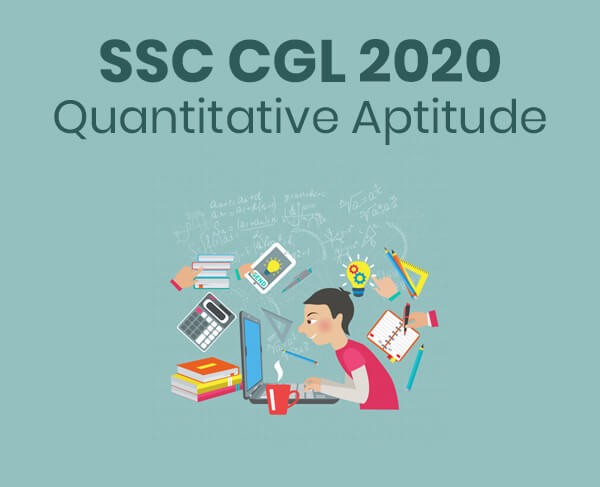Quantitative Aptitude - SSC CGL 2020 - Study24x7Default error msg

New to Study24x7 ?

# Quantitative Aptitude - SSC CGL 2020

Updated on 25 March 2020SSC Preparation Strategies & S
Updated on 25 March 2020In this article, I am going to explain some of the quantitative aptitude topics from the SSC CGL 2020 syllabus.## Simplification

The simplification topic must have a holistic SSC CGL preparation strategy because it includes the application of multiple individual topics in it like fractions, square of a number, algebraic identities, LCM and HCF, etc.

## Basic Rule: “VBODMAS”

This rule is the basis of the simplification question. You must remember the order of simplification through this rule and apply it for the simplification of large and complex numerical expressions.

V is forvirnaculum” or bar represented by(        ).B is for ‘brackets’. Here the Order of precedence for brackets is ( ), { }, [ ].

O is ‘order ’, defines the power or root in the numerical expression.

D is ‘division’.

M is ‘multiplication’.

S is ‘subtraction’.

Example: Solve the numerical expression and find the value of x=?

5(25/5) + 3(27/9) = 5(40/5)-x

Solution: Apply the VBODMAS RULE on the given numerical Expression

5(5) + 3(3) =5(8)-x

25+9=40-x

34=40-x

X=40-34

X= 6

## Interest

The Interest topic of the CGL 2020 quantitative aptitude syllabus includes problems on Simple as well as compound interest.

Remember:

Interest = Amount – Principal

## Basic Formula on Simple Interest:

SI = (PXRXT)/100

Example: A person has borrowed a sum of Rs 25000 from the bank for a period of 3 years. After 3 years he has paid Rs 27250 to the bank. Calculate the rate of simple interest at which the bank has lent the money to the person.

Solution:

Principal (P) =Rs  25000

Amount (A) =Rs  27250

Time (T) = 3 Years

Rate of Interest (R) =?

SI= Amount- Principal

SI= 27250-25000

= Rs 2250

SI= (P*R*T)/100

2250= (25000*R*3)/100

225000= 75000*R

R= 225000/75000

R= 3%

## Basic Formulas on Compound Interest

Amount= P* (1+R/100) T

Example: What will be the compound interest on Rs 10000 for 1 year, if the rate of interest is calculated at 24% per annum?

Solution:

Principal (P) = Rs 10000

Rate of interest(R) = 24%

Time (T) = 1 Year

Compound Interest (CI) =?

Amount = P* (1+R/100) T

= 10000*(1+ 24/100)1

= 10000* (1+0.24)1

= 10000* 1.24

= 12400

Compound Interest= Amount- Principal

= 12400- 10000

= Rs 2400

## Averages

The middle value of a given data set represents the average value of that data set.

Basics:

Average= (Two middle terms of series)/2

Average= Middle Term of the series

## Basic Formula

Average= Sum of all values of observational set Number of values in observational set

Example: A person has received Rs 6434, Rs 7231, Rs 6856, Rs 6927 and Rs 6562 for the 5 months. How much money he will get in the 6th month to make an average of Rs 6500?

Solution: Let the money received in the 6th month= x

Sum of the money received in 5 months

= 6434+ 7231 + 6856 + 6927+ 6562

= 34010

Average of 6 month = (34010+x)/ 6

6500* 6 = 34010+ x

39000 = 34010+ x

x = 39000-34010

= Rs 4990

All The Best To All The SSC CGL 2020 Aspirants !

Write a comment...Trending ArticlesBy CBSE CLASS 8By Edubabble LLPBy CBSE CLASS 7By CBSE CLASS 10
Related PostsServicesNeed Some Help!Connect